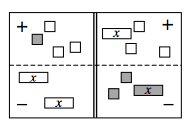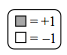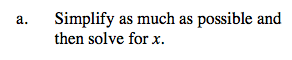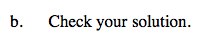### Home > CAAC > Chapter 4 > Lesson 4.1.1 > Problem4-5

4-5.Use the eTool to manipulate the Algebra tiles to help you with this problem.
Click the link at right for the full version of the eTool: AC 4-5 HW eTool

Be sure to know how to get this equation.

1 + (−3) − (−2x) = (−x) + (−3) − 2 − (x)Change all subtraction to addition and combine like terms.

Move the variable to one side of the equation.

1 + (−3) + 2x = (−x) + (−3) + (−2) + (−x)

−2 + 2x = −2x + (−5)

−2 + 2x = −2x + (−5)
+2 +2

2x = −2x + (−3)

2x = −2x + (−3)
+2x +2x

4x = −3

x = −0.75Insert the number you got for x into your original equation to make sure it is correct.